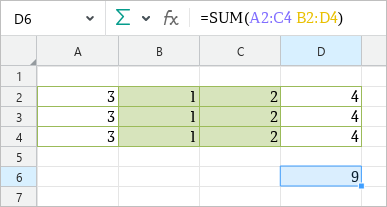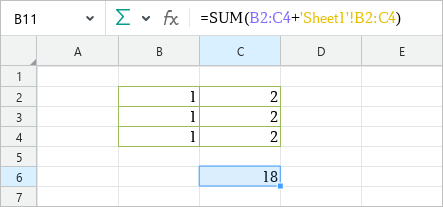Reference operators are used to describe references to cell ranges. It is possible to refer to cells in the current sheet as well as cells in other sheets.

Reference operator

Meaning

Example

:

A range operator.

Creates a reference to a range of cells. The operator is placed between the first and the last cell of the range.

The operator can be used to combine cell ranges. In this case it returns the range between the upper left and lower right cells, including these cells.

=SUM(В2:C4)

=SUM(A1:B1:C4:D4) – in this example, the sum of all cells between and including A1 and D4 is calculated.

Space

Intersection operator. Creates a reference to the cells located at the intersection of the specified ranges.

=SUM(A2:C4 B2:D4)

'sheetname'!

Creates a reference to a cell or range of cells in another sheet of the same spreadsheet.

=SUM(B2+'Sheet2'!В2)

=SUM(B2:C4+'Sheet2'!В2:C4)

'sheetname n:sheetname m'!

Creates a reference to the same cell on several sheets of the current spreadsheet (a 3D reference).

=SUM('January_2019:December_2019'!A1) – in this example, the sum of all A1 cells on all sheets within the specified range is calculated.

When entering formulas, you can select the cell range to avoid entering it manually.Range operatorIntersection operatorReference to a range of cells in another sheet

Was the material useful?
Yes
No
Users found this material useful: 0 из 0